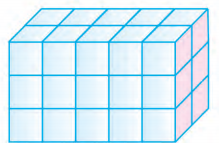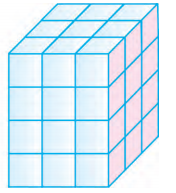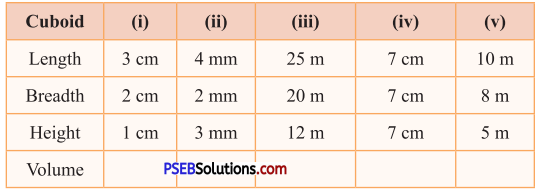# PSEB 5th Class Maths Solutions Chapter 9 Volume Ex 9.1

Punjab State Board PSEB 5th Class Maths Book Solutions Chapter 9 Volume Ex 9.1 Textbook Exercise Questions and Answers.

## PSEB Solutions for Class 5 Maths Chapter 9 Volume Ex 9.1

1. Find the volume of the following by counting the number of cubes.

Question 1.Volume = _________
Solution:
Volume = 4 cm3

Question 2.Volume = _________
Solution:
Volume = 30 cm3Question 3.Volume = _________
Solution:
Volume = 36 cm3

2. Complete the table :Solution:
(i) 6 cm3
(ii) 24 mm3
(iii) 6000 m3
(iv) 343 cm3
(v) 400 m3

3. Find the volume of cube whose side (edge) is :

Question 1.
6 cm
Solution:
Side (edge) of cube = 6 cm
Volume of cube = side × side × side
= 6 cm × 6 cm × 6 cm
= 216 cm3Question 2.
8 m
Solution:
Side of cube = 8 m
Volume of cube = side × side × side
= 8m × 8m × 8m
= 512 m3

Question 3.
15 mm
Solution:
Side of cube = 15 mm
Volume of cube = side × side × side
= 15 mm × 15 mm × 15 mm
= 3375 mm3

Question 4.
21 m
Solution:
Side of cube = 21m
Volume of cube= side × side × side
= 21 m × 21 m × 21 m
= 9261 m3

4. Find the volume of cuboid whose length, breadth and height is as follows :

Question 1.
9 cm, 6 cm, 3 cm
Solution:
Length of cuboid = 9 cm
Breadth of cuboid = 6 cm
Height of cuboid = 3 cm
Volume of cuboid = Length × Breadth × Height
= 9 cm × 6 cm × 3 cm
= 162 cm3Question 2.
12 mm, 9 mm, 4 mm
Solution:
Length of cuboid = 12 mm
Breadth of cuboid = 9 mm
Height of cuboid = 4 mm
Volume of cuboid = Length × Breadth × Height
= 12 mm × 9 mm × 4 mm
= 432 mm3

Question 3.
15 m, 13 m, 12 m
Solution:
Length of cuboid = 15 m
Breadth of cuboid = 13 m
Height of cuboid = 12 m
Volume of cuboid = Length × Breadth × Height
= 15 m × 13 m × 12 m
= 2340 m3

Question 4.
22 mm, 16 mm, 12 mm
Solution:
Length of cuboid = 22 mm
Breadth of cuboid = 16 mm
Height of cuboid = 12 mm
Volume of cuboid = Length × Breadth × Height
= 22 mm × 16 mm × 12 mm
= 4224 mm3

Question 5.
25 m, 23 m, 21 m.
Solution:
Length of cuboid = 25 m
Breadth of cuboid = 23 m
Height of cuboid = 21 m
Volume of cuboid = Length × Breadth × Height
= 25 m × 23 m × 21 m
= 12075 m3Question 5.
A chalk box is 8 cm long, 6 cm broad and 10 cm high. Find the volume of the box.
Solution:
Length of the chalk box = 8 cm
Breadth of the chalk box = 6 cm
Height of the chalk box = 10 cm
Volume of the chalk box = Length × Breadth × Height
= 8 cm × 6 cm × 10 cm
= 480 cm3

Question 6.
A cardboard box is 50 cm long, 40 cm wide and 24 cm high. Find the volume of the box.
Solution:
Length of the cardboard box = 50 cm
Breadth of the cardboard box = 40 cm
Height of the cardboard box = 24 cm
Volume of the cardboard box = Length × Breadth × Height
= 50 cm × 40 cm × 24 cm
= 48000 cm3

Question 7.
Jashan’s tiffin box is 15 cm long, 10 cm wide and 8 cm high and Gurwinder’s tiffin box is 12 cm long, 10 cm wide and 10 cm high. Find the volume of both. Whose tiffin box has more volume ?
Solution:
Jashan :
Length of the tiffin box = 15 cm
Breadth of the tiffin box = 10 cm
Height of the tiffin box = 8 cm
Volume of the tiffin box = Length × Breadth × Height
= 15 cm × 10 cm × 8 cm
= 1200 cm3

Gurwinder :
Length of the tiffin box = 12 cm
Breadth of the tiffin box = 10 cm
Height of the tiffin box = 10 cm
Volume of the tiffin box = Length × Breadth × Height
= 12 cm × 10 cm × 10 cm
= 1200 cm3
Their tiffin boxes have equal volumes.Question 8.
Find the volume of 25 cuboidal boxes with dimensions 12 cm long, 9 cm wide and 6 cm high each.
Solution:
Length of the cuboidal box =12 cm
Breadth of the cuboidal box = 9 cm
Height of the cuboidal box = 6 cm
Volume of the cuboidal box = Length × Breadth × Height
= 12 cm × 9 cm × 6 cm
= 648 cm3
Volume of 25 cuboidal boxes
= 648 × 25 cm3
= 16200 cm3

Question 9.
There are two types of powder boxes available in the market. One is of cubical shape with side 8 cm and other is of cuboidal shape with length 15 cm, breadth 8 cm and height 4 cm. Which box has more powder and how much? If both have same prices then which box will you prefer?
Solution:
Side of the cubical box = 8 cm
Volume of the cubical box = side x side x side
= 8 cm × 8 cm × 8 cm
= 512 cm3
Length of the cuboidal box = 15 cm
Breadth of the cuboidal box = 8 cm
Height of the cuboidal box = 4 cm
Volume of the cuboidal box = Length × Breadth × Height
= 15 cm × 8 cm × 4 cm
= 480 cm3
Cubical box has more powder
= 512 cm3 – 480 cm3
= 32 cm3
I will prefer cubical box.Question 10.
How many bricks of size 24 cm long, 12 cm wide and 8 cm thick are required for* making a wall of 12 m long, 3 m high and 24 cm thick?
Solution:
Length of the wall = 12 m
= 12 × 100 cm
= 1200 cm
Height of the wall = 3 m
= 3 × 100 cm
= 300 cm
Breadth of the wall = 24 cm
Volume of the wall = Length × Breadth × Height
= 1200 cm × 24 cm × 300 cm
= 8640000 cm3
Length of each brick = 24 cm
Breadth of each brick = 12 cm
Height of each brick = 8 cm
Volume of each brick = Length × Breadth × Height
= 24 cm × 12 cm × 8 cm
= 2304 cm3
Number of bricks required
= $$\frac{8640000 \mathrm{~cm}^{3}}{2304 \mathrm{~cm}^{3}}$$
= 3750 bricks.

Question 11.
The length, breadth and height of a biscuit packet is 15 cm, 9 cm and 6 cm respectively. If a packet has 30 biscuits then Bind the volume of each biscuit.
Solution:
Length of the packet = 15 cm
Breadth of the packet = 9 cm
Height of the packet = 6 cm
Volume of the packet = Length × Breadth× Height
= 15 cm × 9 cm × 6 cm
= 810 cm3
Total number of biscuits in the packet = 30
Volume of each biscuit = $$\frac{810 \mathrm{~cm}^{3}}{30}$$
= 27 cm3Question 12.
One trolley is full of bricks. It is 4 m long, 2 m wide and 60 cm deep. One brick is of size 20 cm x 10 cm x 6 cm. How many bricks are there in the trolley ?
Solution:
Length of the trolley
= 4m = 4 × 100 cm = 400 cm
= 2 m = 2 × 100 cm = 200 cm
Depth (Height) of the trolley = 60 cm
Volume of the trolley = Length × Breadth × Depth
= 400 cm × 200 cm × 60 cm
= 4800000 cm3
Length of the brick = 20 cm
Breadth of the brick = 10 cm
Height of the brick = 6 cm
Volume of the brick = Length × Breadth × Height
= 20 cm × 10 cm × 6 cm
= 1200 cm3
Number of bricks in the trolley
= $$\frac{4800000}{1200}$$ cm3
= 4000.

13. Fill in the blanks :

Question 1.
Volume of cube = __________ × __________ × ________
Solution:
Side × Side × Side

Question 2.
Volume of cuboid = __________ × __________ × ________
Solution: